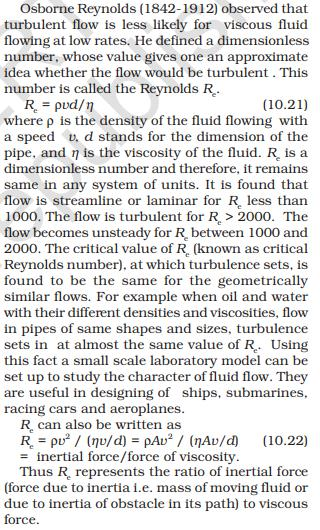NEET  >  Test: Non-Ideal Fluids

# Test: Non-Ideal Fluids - NEET

Test Description

## 10 Questions MCQ Test Physics Class 11 - Test: Non-Ideal Fluids

Test: Non-Ideal Fluids for NEET 2023 is part of Physics Class 11 preparation. The Test: Non-Ideal Fluids questions and answers have been prepared according to the NEET exam syllabus.The Test: Non-Ideal Fluids MCQs are made for NEET 2023 Exam. Find important definitions, questions, notes, meanings, examples, exercises, MCQs and online tests for Test: Non-Ideal Fluids below.
Solutions of Test: Non-Ideal Fluids questions in English are available as part of our Physics Class 11 for NEET & Test: Non-Ideal Fluids solutions in Hindi for Physics Class 11 course. Download more important topics, notes, lectures and mock test series for NEET Exam by signing up for free. Attempt Test: Non-Ideal Fluids | 10 questions in 10 minutes | Mock test for NEET preparation | Free important questions MCQ to study Physics Class 11 for NEET Exam | Download free PDF with solutions
 1 Crore+ students have signed up on EduRev. Have you?
Test: Non-Ideal Fluids - Question 1

### The viscosity of liquids _________ with increase in temperature.

Detailed Solution for Test: Non-Ideal Fluids - Question 1

The viscosity of liquids decreases with increase in temperature. The cohesive forces between molecules of liquids decrease as temperature increases. At high temperature the molecules of liquids have high energy and overcome strong cohesive forces and move freely. Hence, viscosity of liquids decreases with increase in termperature.

Test: Non-Ideal Fluids - Question 2

### The terminal velocity of a steel ball of 4 mm in diameter falling through a glycerin of viscosity 3.3 poise, given relative density of steel is 8, relative density of glycerin is 1.3 is

Test: Non-Ideal Fluids - Question 3

### As the velocity of a body increases, the viscous force on it

Detailed Solution for Test: Non-Ideal Fluids - Question 3

Drag acts as an opposing force to acceleration. Drag will never increase velocity, but whilst the forces acting to move the object are greater than the drag or resistive forces then the object will continue to accelerate, thus increasing velocity. Simplified, drag is caused by the object colliding with air particles. As the object moves faster, the object will come into contact with more particles and this will increase the effect of drag. Drag decreases acceleration and therefore velocity, but an increase in velocity will increase drag forces.

Test: Non-Ideal Fluids - Question 4

A value of Reynold’s number _________ indicates turbulence.

Detailed Solution for Test: Non-Ideal Fluids - Question 4Test: Non-Ideal Fluids - Question 5

The viscosity of gases ________ with increase in temperature.

Detailed Solution for Test: Non-Ideal Fluids - Question 5

According to kinetic theory of gases viscosity in gases increases with increase in temperature because increase in kinetic energy will increase the frequency of collision between the molecules.

Test: Non-Ideal Fluids - Question 6

Terminal velocity of a spherical body of density ρ and radius r moving through a liquid of density ρ’ is given by

Test: Non-Ideal Fluids - Question 7

The relative velocity between two parallel layers of water is 10 cm/s and the perpendicular distance between them is 0.1 cm. The velocity gradient is

Detailed Solution for Test: Non-Ideal Fluids - Question 7

dv = 10 cm/s
dx = 0.1 cm
dv/dx = 10/0.1 = 100cm/s cm

Test: Non-Ideal Fluids - Question 8

Force of viscosity acting on a spherical body of radius r moving with velocity v through a fluid of viscosity η is given by

Detailed Solution for Test: Non-Ideal Fluids - Question 8

Stoke’s Law calculates the drag force on the sphere as it travels through a fluid.
F = 6 πrηv

Test: Non-Ideal Fluids - Question 9

The quantity which decides the nature of flow of a viscous liquid through a pipe is known as

Detailed Solution for Test: Non-Ideal Fluids - Question 9

The Reynolds Number (Re) is proportional to the ratio between the momentum of a flowing fluid and its viscosity. The lower Re, the more viscosity governs the flow behavior, so the more it sticks to a surface, and the flow is laminar. The higher the Re, the more its velocity governs, so the less it sticks to surface and the flow is turbulent. Laminar flow is straight lines. Turbulent flow is eddies and swirling vortexes.

Test: Non-Ideal Fluids - Question 10

Range of Reynold’s number for the laminar flow of a liquid is

## Physics Class 11

130 videos|483 docs|210 tests
Information about Test: Non-Ideal Fluids Page
In this test you can find the Exam questions for Test: Non-Ideal Fluids solved & explained in the simplest way possible. Besides giving Questions and answers for Test: Non-Ideal Fluids, EduRev gives you an ample number of Online tests for practice

## Physics Class 11

130 videos|483 docs|210 tests

### How to Prepare for NEET

Read our guide to prepare for NEET which is created by Toppers & the best Teachers(Scan QR code)